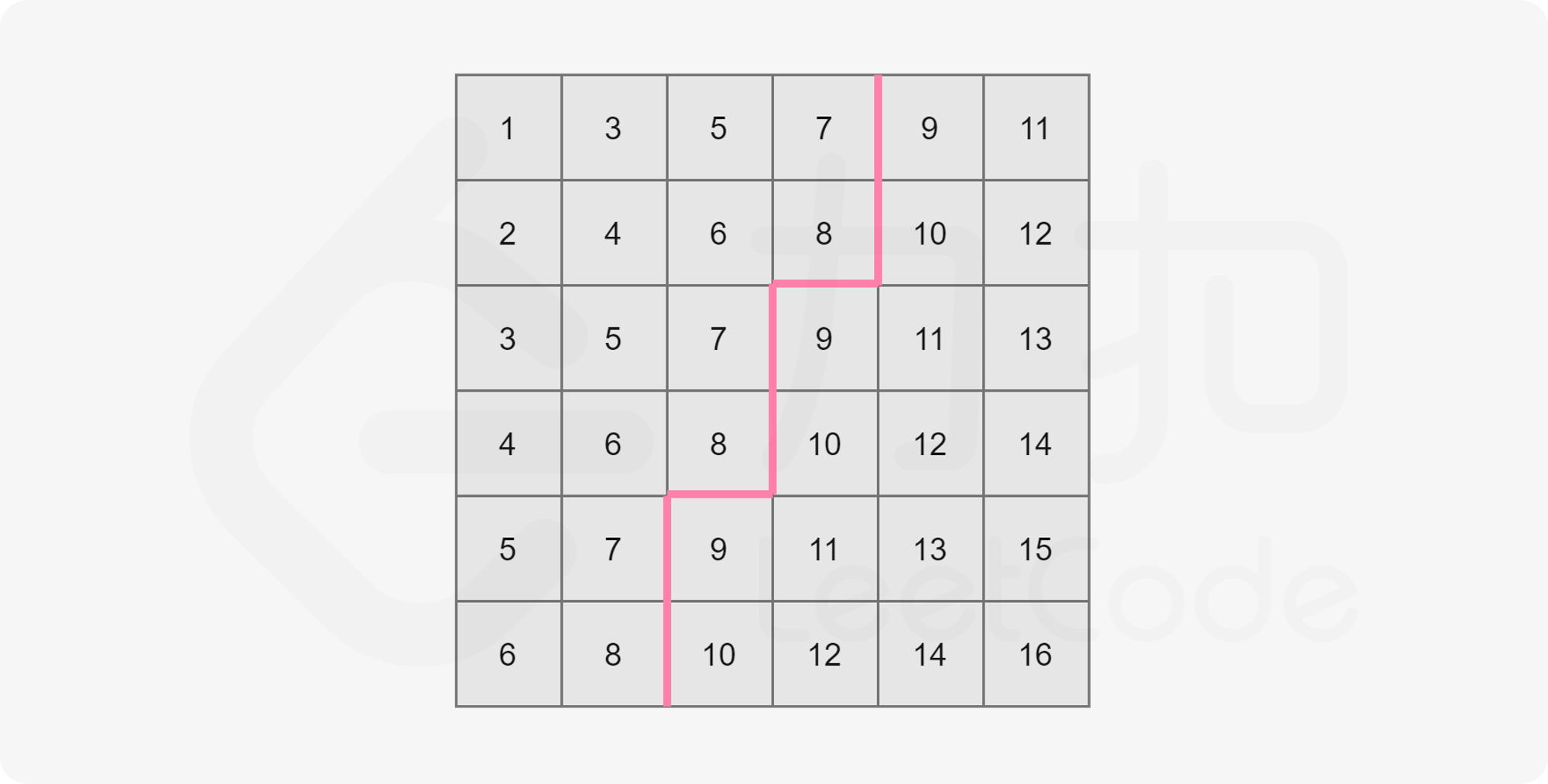# Leetcode 378 - Kth smallest element in a sorted matrix

Note:

•• Why can we use binary search on this question? Especially, how do we know mid = (low + right) / 2 is indeed in the matrix?
• Well, by resizing the window gradually, we are always sure that the result between [low, high].
• When the loop terminates, we are sure low (or high coz they are equal at that moment) is the answer.
• Detail:
• In order not to traverse the whole matrix every time to count val <= k, count elements starting from the end of each col.

Question:

Given an n x n matrix where each of the rows and columns is sorted in ascending order, return the kth smallest element in the matrix.

Note that it is the kth smallest element in the sorted order, not the kth distinct element.

You must find a solution with complexity better than O(n2).

Example:

Code: